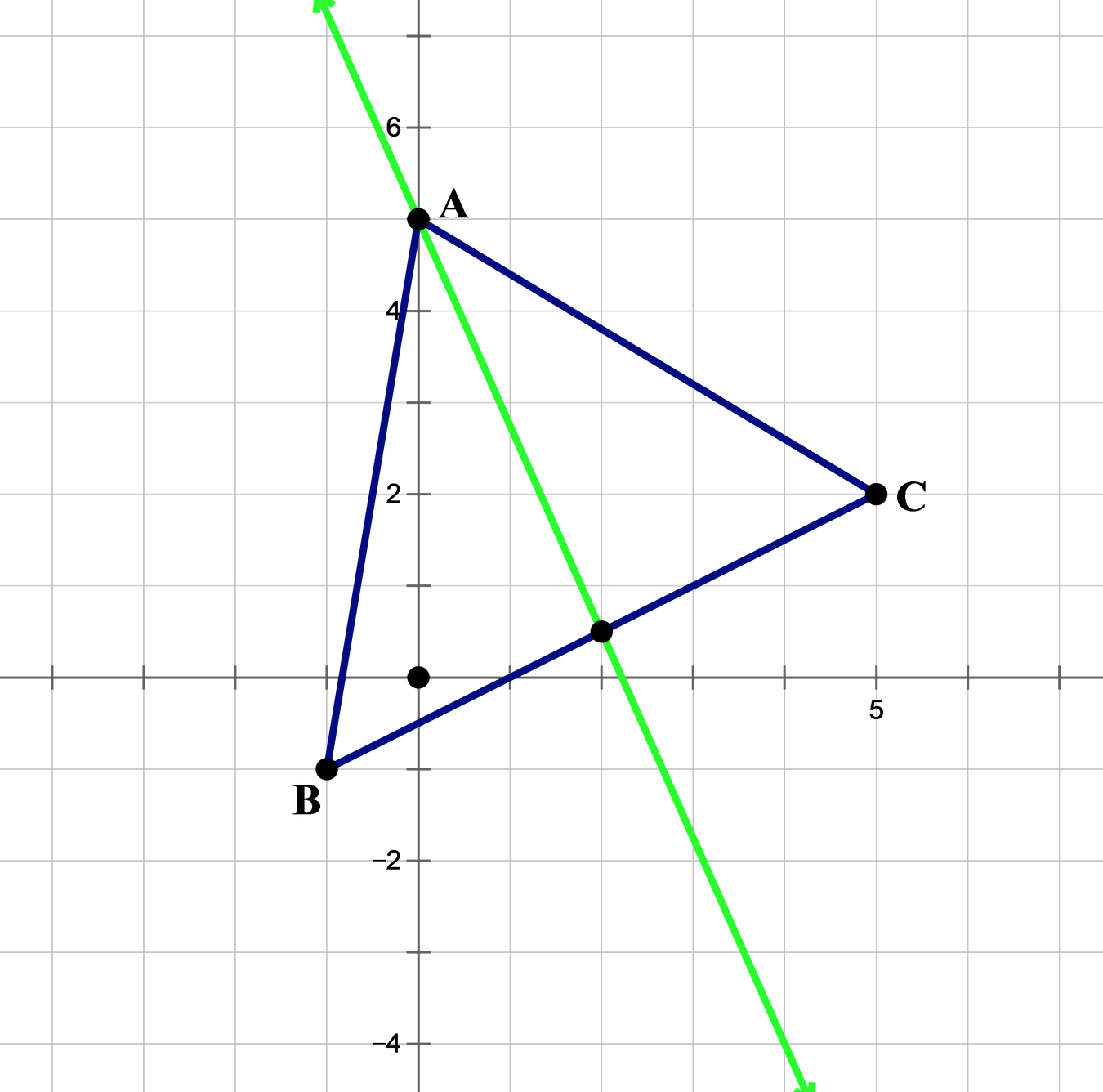# GMAT & MBA Admissions BlogGMAT Data Sufficiency problems present you with some initial information and a question, followed by two statements.  You have to decide whether the information contained in each statement is sufficient, when combined with the initial information, to give a definite answer to the question.  In this case, a definite answer means being able to answer either “definitely yes” or “definitely no” to the question.  If you can only answer “definitely maybe” then the statement is not sufficient by itself.  If neither statement is sufficient by itself to give a definite answer, you then evaluate whether the two statements taken together are sufficient to find a definite answer.  Some questions involve solving for a value; for these questions, a statement is sufficient if it allows you to solve for one, but no more than one, value.

In case you haven’t seen a Data Sufficiency question before, here’s an example:

If x is even and y is a positive integer, is the sum of x and y an odd integer?

1)   x is a negative integer

2)   the square of y is an even integer

Notice that since we already know that x is even, the only way for the sum of x and y to be odd is for y itself to be odd.  So we can rephrase the question as “Is y an odd integer?”

Statement 1 is irrelevant.  The fact that x is negative tells us nothing about whether y is odd or even.  So statement 1 is insufficient to answer the question.

Statement 2 is a different story.  If y-squared is an even integer, that tells us that y itself is an even integer.  Therefore we can answer “definitely not” to the question “is y an odd integer”, and statement 2 by itself is sufficient.

Here’s a list of tips to help you in solving GMAT Data Sufficiency questions:

1. It’s helpful if you can restate the question in simpler terms (as we did in the example above).
2. Plugging in example numbers is also helpful.  However, be careful not to make unwarranted assumptions about the kind of numbers you can plug in.  For example, if the initial information in a question specifies that the quantity n is less than zero, be careful not to assume that n is necessarily an integer.  Integers probably jump most readily to mind when making up examples, but data sufficiency questions often deal with non-integer values as well.
3. Quickly look at both statements and try to evaluate which one will be easier to analyze.  If statement 2 looks easier than statement 1, then test statement 2 first.
4. If the first statement you test seems insufficient, remember to “forget” that statement when you move on to test the other statement.   You have to test each statement alone for sufficiency before you test whether the statements are sufficient when combined together.
5. On the other hand, if you feel really stuck on a Data Sufficiency question, go ahead and combine the statements to see if they’re sufficient together to answer the question.  HOWEVER, if they are sufficient together, you STILL need to look carefully and see if there’s any way to take just one of the statements by itself and find a definite answer to the question.  This is a risky technique if you don’t have enough practice in separating the statements in your thoughts.  But if you’re careful, it can pay off by making it easier to see how to restate the question or find the key connection between the statements and the initial information.

Topics: math skills, GMAT